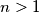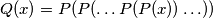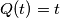### IMO Shortlist 2006 problem N4

Kvaliteta:
Avg: 0,0
Težina:
Avg: 7,0
Let$P(x)$ be a polynomial of degree$n > 1$ with integer coefficients and let$k$ be a positive integer. Consider the polynomial$Q(x) = P(P(\ldots P(P(x)) \ldots ))$, where$P$ occurs$k$ times. Prove that there are at most$n$ integers$t$ such that$Q(t) = t$.
Izvor: Međunarodna matematička olimpijada, shortlist 2006